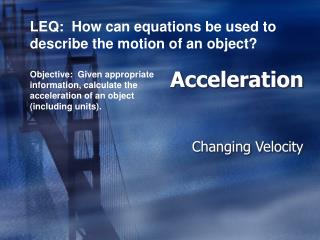DownloadDownload PresentationAcceleration

# Acceleration

Télécharger la présentation## Acceleration

- - - - - - - - - - - - - - - - - - - - - - - - - - - E N D - - - - - - - - - - - - - - - - - - - - - - - - - - -
##### Presentation Transcript

1. LEQ: How can equations be used to describe the motion of an object? Objective: Given appropriate information, calculate the acceleration of an object (including units). Acceleration Changing Velocity

2. Acceleration • Galileo developed the concept of acceleration in his experiments with inclined planes. • http://www.teachersdomain.org/resources/lsps07/sci/phys/maf/ballincline/index.html • http://www.msad49.org/ljhs/WonWorld/Inter2/lsps07_vid_ballincline/lsps07_vid_ballincline.html

3. Acceleration • Galileo defined acceleration as the rate of change of velocity • Acceleration = • a = = Change of velocity Time interval vvf - vi t t

4. Acceleration • There are three ways a velocity can change: • Increase velocity (speed up) • Decrease velocity (slow down) • Change directions (turn) • All three of these changes in velocity are forms of acceleration.

5. Acceleration • A car’s speed increases from 30 km/hr to 60 km/hr in 5 sec. What is its acceleration?

6. Acceleration • A runner starts from rest and reaches a speed of 6 m/s in 2 sec. What is his acceleration?

7. Acceleration • A car starting from rest reaches a speed of 40 km/hr in 2 sec. What is its acceleration?

8. Acceleration • A car traveling 60 km/hr stops in 3 sec. What is its acceleration?

9. Acceleration • A car starts from rest and reaches a speed of 125 km/hr in 5 sec. What is its acceleration?